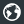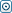Forex Stock Exchange Forum
Would you like to react to this message? Create an account in a few clicks or log in to continue.

# I-Regression a VertexFX client-side indicatorOmSaiTech
Number of messages : 62
Points : 2186
Date of Entry : 2016-05-08
Year : 42
Residence Country : India##I-Regression a VertexFX client-side indicator

Wed Jun 01, 2016 9:59 am

I Regression is a powerful Vertex client-side indicator used to calculate and display regression data for a specific chart.
It is used to identify sideways and trending markets using the First degree regression, and the market cycles using the second and third degree

regressions.

It has three modes of operation, configured based on the DEGREE input parameter –

Number 1 Mode is Linear Regression Mode – As the name implies, it displays three lines, the middle GREEN line which is the linear regression line,  a

yellow line each above and below the middle GREEN line. The two YELLOW lines are K S T D times the standard deviation away from the middle

GREEN line. When the prices are restricted within the outside YELLOW lines, it is clearly that volatility is below K S T D number of standard

deviations.
Hence, breakout trades can be placed beyond these two YELLOW lines.

Similarly the Number 2 Mode is Parabolic Regression Mode –The Parabolic Regression Mode is the second  degree regression mode using second

power filters to calculate the regression lines instead of first power (linear) regression.
Breakout trades can be placed above and below the YELLOW lines.

Similarly the Number 3 Mode is Polynomial – The Polynomial Regression Mode is the Third degree regression mode using Third  power filters to

calculate the regression lines.

Breakout trades can be placed above and below the YELLOW lines.
Permissions in this forum:
You cannot reply to topics in this forum